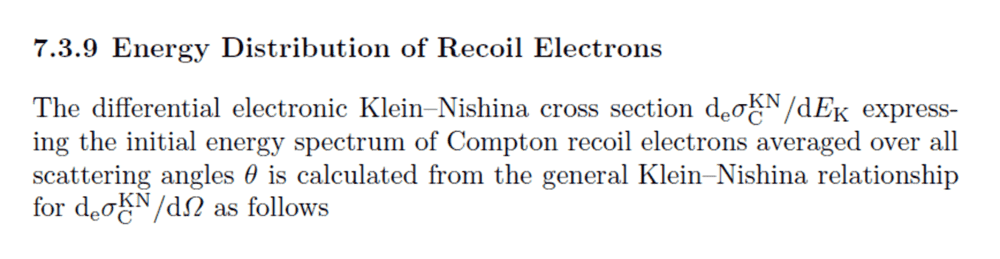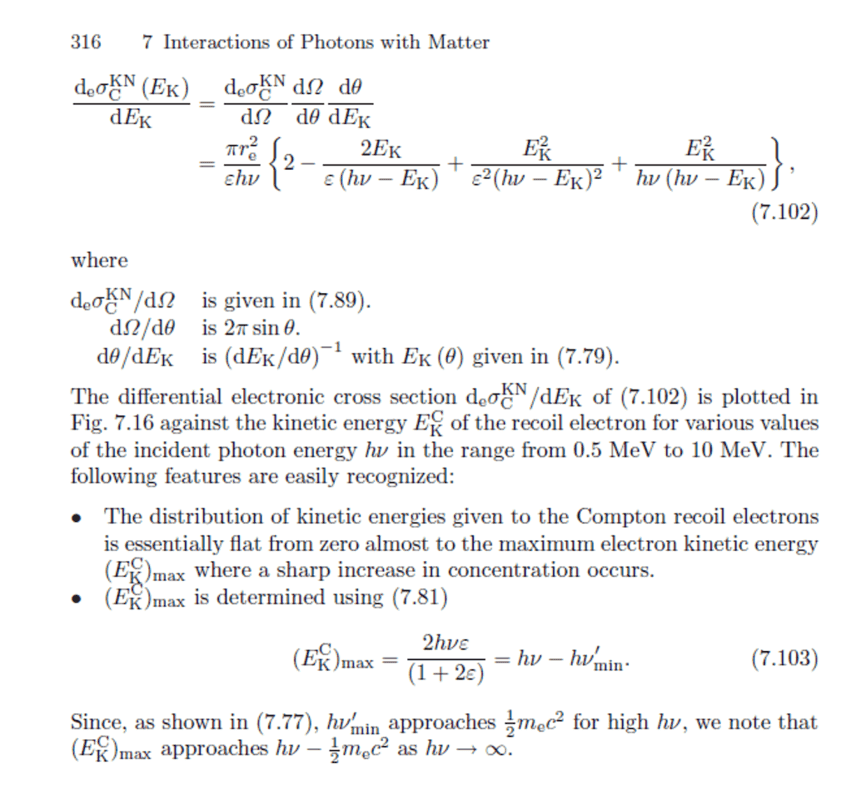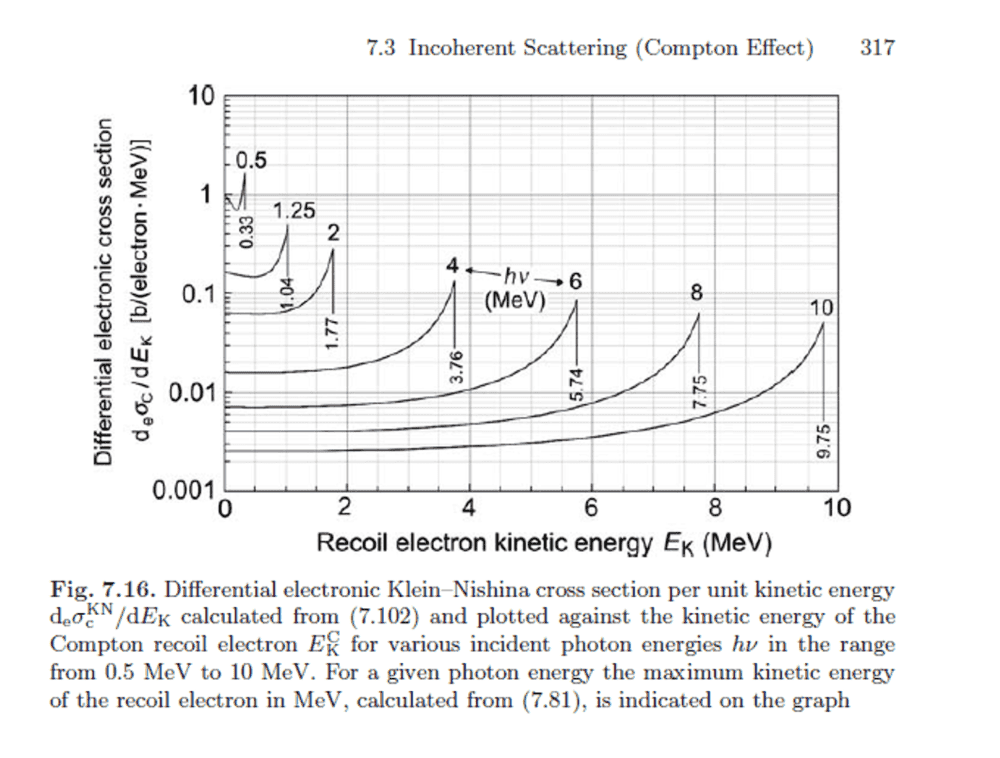# Compton Recoil and the Electron Differential Cross Section

• A

TL;DR Summary
I'm trying to reconcile the differential cross sections for Compton recoil electron energies with that of photons scattered into a certain solid angle
Hi folks,

My understanding of the Compton Effect is that maximum energy transfer to the electron takes place when the photon scattering angle is 180 degrees.

For the following please reference Evans "The Atomic Nucleus" :
https://library.psfc.mit.edu/catalog/online_pubs/books/evans_atomic_nucleus.pdf

Page 683 (697 of the PDF): Eq. 2.8 and Fig 2.3 (polar plot) show that for photons of higher incident energy, the differential cross section shows a tendency to forward scatter (photon scattering angle closer to zero degrees)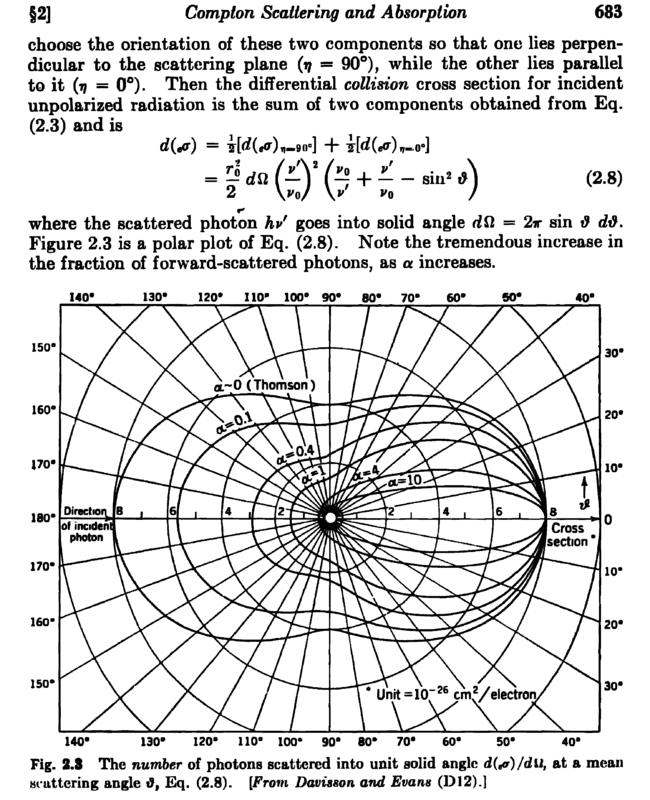Therefore, it seems that high-energy photons are more likely to transfer *little* energy to electrons.

Now Eq. 5.2 and Fig 5.1 for the energy spectrum of recoil electrons show that the differential cross section for scattering electrons into a certain recoil energy interval is highest at the Compton Edge (i.e. 180 degree scattering)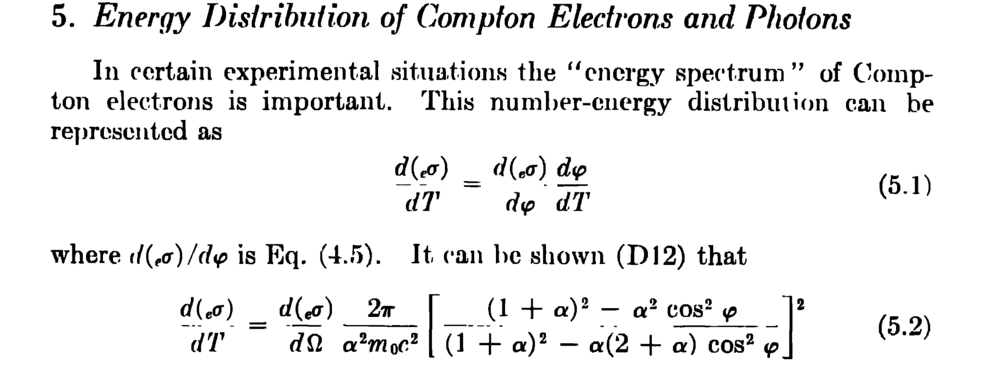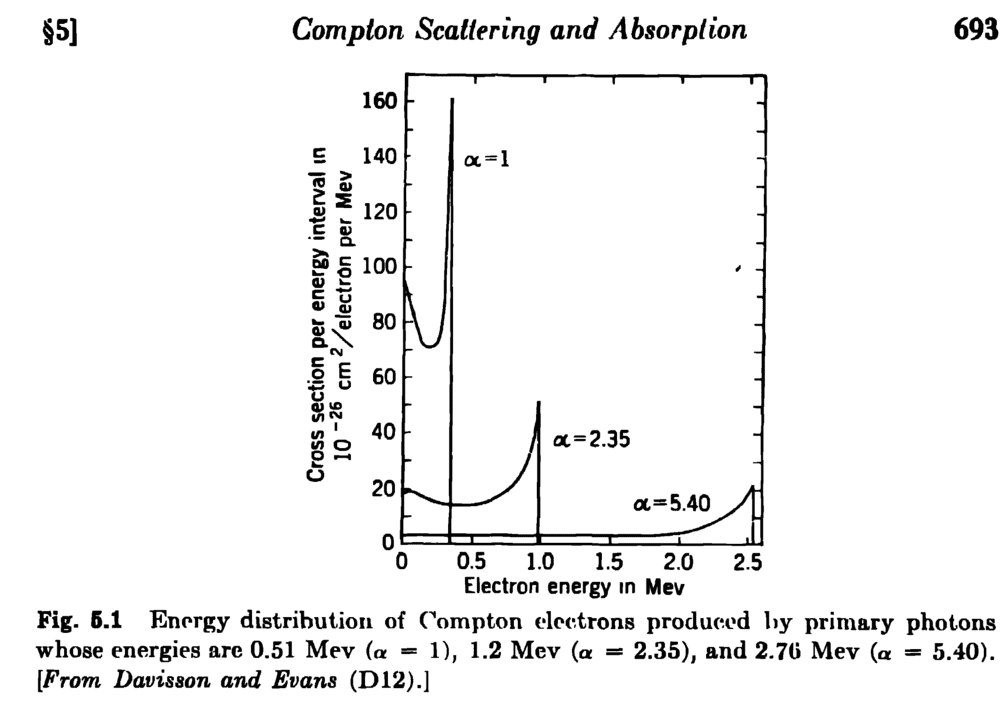These two differential cross sections seem contradictory to me. dsigma/dOmega tells me that high-energy photons are more likely to scatter at zero degrees but dsigma/dT tells me that scattering will most likely give recoil-electron energies at the Compton edge, which implies a photon scattering angle of 180 degrees. What am I missing here? T

Well, most of the events for the ##\alpha=1## curve are likely at lower energies and therefore larger scattering angles than the peak at the Compton edge. That's a pretty narrow peak.

Yes but the alpha=1 curve in the polar plot appears to be forward peaked (photon scattering angle near zero - i.e. little energy transferred to the electron) - it's like the two plots are contradictory.

Not at all. The total number of scattering events in a range of angles or a range of energies is given by the integral of the corresponding differential cross section times the appropriate differential (and times the incident flux). In the case of angle this factor is ##2\pi\sin\theta## well known to be 0 at 0 and 180. I can't recall what the factor would be in energy. This is likely given in the reference you provided. In energy the number of events under the narrow Compton edge less that those scattered at higher angles to my eye assuming a differential weight of 1.

Also, kinematically scattering angle is a function of energy transfer and visa versa. Equations 5.1 and 5.2 appear to be discussing this.

Also, kinematically scattering angle is a function of energy transfer and visa versa. Equations 5.1 and 5.2 appear to be discussing this.
Yes well this is what I was thinking. Considering that even for alpha=1 the polar plot shows the cross section at near-zero scattering angle (low energy transfer) to be several times larger than for 180-deg scattering. That is why I did not expect the recoil energy to peak at the Compton Edge (180-deg scattering/max energy transfer) - But - you are right, I did not consider that I need to integrate. Thanks for your time on this.

•berkeman
Here is another reference (Podogorsak (2010)) that also sheds light on this:

So my confusion was focused on how the differential cross section w.r.t. to solid angle tends toward forward scattering (i.e. low energy transfer to the recoil electrons) - but the differential cross section w.r.t. recoil-electron energy peaks at the maximum possible energy. It seems one needs to consider the other parts of this cross section shown below (7.102), the derivative of solid angle w.r.t. photon scattering angle and the derivative of the photon scattering angle w.r.t. the recoil-electron energy. These two terms must peak at the maximum energy to count the effect of the cross section w.r.t. solid angle.